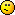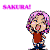Discussion about math, puzzles, games and fun.   Useful symbols: ÷ × ½ √ ∞ ≠ ≤ ≥ ≈ ⇒ ± ∈ Δ θ ∴ ∑ ∫  π  -¹ ² ³ °

You are not logged in.

## #1 2006-02-21 10:12:42

se7en
Member
Registered: 2005-12-25
Posts: 28

### a cool maths problem I saw in the latest issue of Hugi

I have a really cool maths problem for you! Pick two random points on the arc of a circle. What's the probability that the straight line that connects these two points is longer than the radius of the circle?

I'm still working on this problem myself. I've been toying around with a lot of different ideas and I've learnt quite a bit about the circle in the process. One thing I tried:

upper semi-circle - lower semi-circle = radius (r)
sqrt(r^2 - x^2) + sqrt(r^2 - x^2) = r
solving for r we get: r = +- 2x/sqrt(3)

if we solve for x we can obtain the values of x for which the upper semi-circle - lower semi-circle = r

therefore, solving for x we get: x = +- r * sqrt(3) / 2

therefore, - r * sqrt(3) / 2 < x < r * sqrt(3) / 2 then sqrt(r^2 - x^2) - -sqrt(r^2 - x^2) > r

now, the number of chords in a circle > radius of the circle (provided the chords are all parallel to the y-axis) = r * sqrt(3) / 2 - -r * sqrt(3) / 2

let the number of chords = n

2 * r * sqrt(3) / 2 = n

r * sqrt(3) = n

that is to say, the number of chords in a circle > radius of the circle = r * sqrt(3) provided the chords are all parallel to the y-axis.

I thought that was really interesting.Now, the probability of an event is defined as the number of outcomes in the event n(E) over the number of outcomes in the sample space n(S). I've managed to determine the number of outcomes in the sample space for this event... it's simply the circumference of the circle * the circumference of the circle, or 4 * pi^2 * r^2. The remaining piece of the puzzle is what n(E) is. I think the answer lies somewhere in the research I've done... I just have to determine the TOTAL number of chords in a circle > the radius of the circle (i.e. the chords are not limited to being parallel to the y-axis). Then you divide that by n(S) = 4 * pi^2 * r^2 and voila! I'm guessing that n(E) contains the expression r^2 somewhere, so the radius cancels out. (Otherwise P(E) - the probability of the event - will contain the variable r, which is obviously undesirable... we want just a number without any variables.)

I'm going to take an educated guess and say that the TOTAL number of chords in a circle > radius of the circle = r * sqrt(3) * 1/2 * circumference of the circle (the reason that we multiply by only HALF the circumference of the circle is that the second half of the circle contains exactly the same chords as the first half... in actual fact, we should multiply by 1/2 * (circumference of the circle - 1/infinity) because the chords at the highest point of the circle are the same as the chords on the lowest part of the circle, but 1/infinity tends to zero so leaving it out of our calculations is still accurate enough for all practical purposes) = r * sqrt(3) * pi * r = sqrt(3) * pi * r^2

If that is true, then

P(E) = n(E) / n(S)
= (sqrt(3) * pi * r^2) / (4 * pi^2 * r^2)
= sqrt(3) / (4 * pi)
= 1,732 / 12.566
= 0.138

But I don't know if this is right or not.I guess I could write a program to test this out.

All in all, quite an interesting (and frustrating) puzzle! Good luck with it! (This somewhat long rant will probably help get you started out, although I wouldn't be surprised if your method of approach differs entirely from mine. Let me know what you come up with!)

Offline

## #2 2006-02-22 04:51:35

espeon
Real MemberRegistered: 2006-02-05
Posts: 2,586

### Re: a cool maths problem I saw in the latest issue of Hugi

probably an even chance

Presenting the Prinny dance.
Take this dood! Huh doood!!! HUH DOOOOD!?!? DOOD HUH!!!!!! DOOOOOOOOOOOOOOOOOOOOOOOOOD!!!!!!!!!!!!!!!!!!!!!!!!!!!!!!

Offline

## #3 2006-02-22 08:57:28

MathsIsFun
AdministratorRegistered: 2005-01-21
Posts: 7,691

### Re: a cool maths problem I saw in the latest issue of Hugi

If I had time I would like to write a program to simulate that. The answer may have π in it.

"The physicists defer only to mathematicians, and the mathematicians defer only to God ..."  - Leon M. Lederman

Offline

## #4 2006-03-13 10:23:27

Ikcelaks
Member
Registered: 2006-03-13
Posts: 8

### Re: a cool maths problem I saw in the latest issue of Hugi

The probability is exactly 2/3.  Actually, this is a very simple puzzle if you attack it right (I got the answer in less than one minute.).

Proof:

Inscribe a regular hexagon inside the circle such that one of the vertices corresponds to one of the randomly chosen points.  Each of the edges of the inscribed hexagon is a cord with the exact same length as the circle's radius (This is obvious because a regular hexagon can be subdivided into six equilateral triangles and, in this case, two of those equilateral edges corresponds to the circles radius and the cord is the third.).

Therefore, the vertices of the hexagon have subdivided the circumference of the circle into six equal segments AND we know that all points that fall within the two circle segments that are adjacent to the vertex on that first random point form cords with it that are less than the circle's radius and all points that are beyond those two adjacent circle segments form cords that are longer than the radius of the circle.  Since four of the six equal length segments aren't adjacent to the original random point.  The probability that the second randomly selected point falls in one of them is 2/3.

Offline

## Board footer

Powered by FluxBB